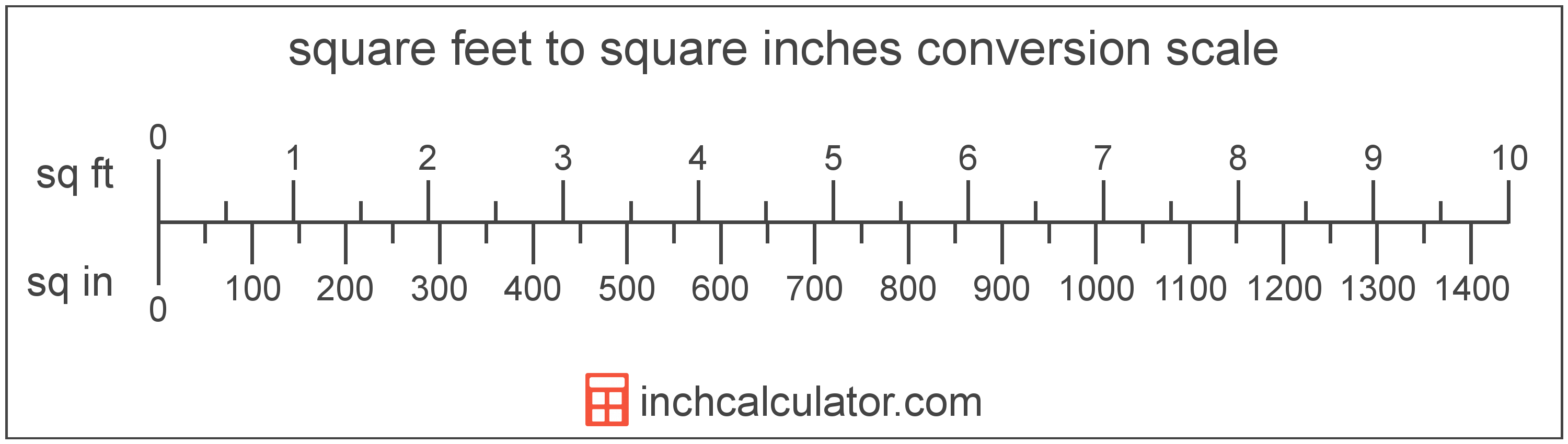# Square Inches to Square Feet Conversion

Enter the area in square inches below to get the value converted to square feet.

Results in Square Feet:144 sq in = 1 sq ft

## How to Convert Square Inches to Square FeetTo convert a square inch measurement to a square foot measurement, divide the area by the conversion ratio.

Since one square foot is equal to 144 square inches, you can use this simple formula to convert:

square feet = square inches ÷ 144

The area in square feet is equal to the square inches divided by 144.

For example, here's how to convert 500 square inches to square feet using the formula above.
500 sq in = (500 ÷ 144) = 3.472222 sq ft

## Square Inches

One square inch is equal to the area of a square with sides that are 1 inch in length.

The square inch is a US customary and imperial unit of area. A square inch is sometimes also referred to as a square in. Square inches can be abbreviated as sq in, and are also sometimes abbreviated as in². For example, 1 square inch can be written as 1 sq in or 1 in².

## Square Feet

One square foot is equal to the area of a square with sides that are 1 foot in length. One square foot is roughly equivalent to 144 square inches.

The square foot is a US customary and imperial unit of area. A square foot is sometimes also referred to as a square ft. Square feet can be abbreviated as sq ft, and are also sometimes abbreviated as ft². For example, 1 square foot can be written as 1 sq ft or 1 ft².

Try our square footage calculator to calculate the area of a space.

## Square Inch to Square Foot Conversion Table

Square inch measurements converted to square feet
Square Inches Square Feet
1 sq in 0.006944 sq ft
2 sq in 0.013889 sq ft
3 sq in 0.020833 sq ft
4 sq in 0.027778 sq ft
5 sq in 0.034722 sq ft
6 sq in 0.041667 sq ft
7 sq in 0.048611 sq ft
8 sq in 0.055556 sq ft
9 sq in 0.0625 sq ft
10 sq in 0.069444 sq ft
20 sq in 0.138889 sq ft
30 sq in 0.208333 sq ft
40 sq in 0.277778 sq ft
50 sq in 0.347222 sq ft
60 sq in 0.416667 sq ft
70 sq in 0.486111 sq ft
80 sq in 0.555556 sq ft
90 sq in 0.625 sq ft
100 sq in 0.694444 sq ft
200 sq in 1.3889 sq ft
300 sq in 2.0833 sq ft
400 sq in 2.7778 sq ft
500 sq in 3.4722 sq ft
600 sq in 4.1667 sq ft
700 sq in 4.8611 sq ft
800 sq in 5.5556 sq ft
900 sq in 6.25 sq ft
1,000 sq in 6.9444 sq ft

## References

1. Merriam-Webster, square foot, https://www.merriam-webster.com/dictionary/square%20foot